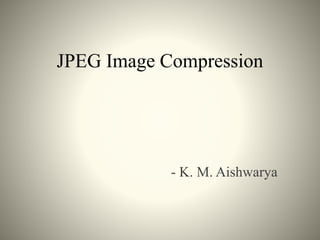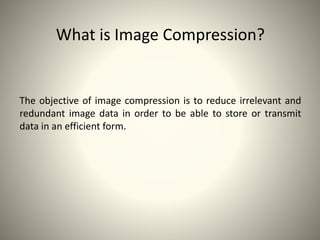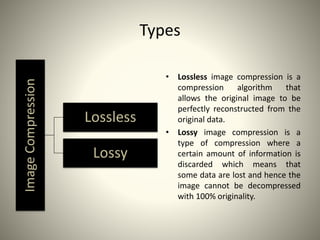Diese Präsentation wurde erfolgreich gemeldet.

# JPEG Image Compression×

1 von 31
1 von 31

# JPEG Image Compression

JPEG Compression Algorithm

JPEG Compression Algorithm

### JPEG Image Compression

1. 1. JPEG Image Compression - K. M. Aishwarya
2. 2. What is Image Compression? The objective of image compression is to reduce irrelevant and redundant image data in order to be able to store or transmit data in an efficient form.
3. 3. TypesImageCompression Lossless Lossy • Lossless image compression is a compression algorithm that allows the original image to be perfectly reconstructed from the original data. • Lossy image compression is a type of compression where a certain amount of information is discarded which means that some data are lost and hence the image cannot be decompressed with 100% originality.
4. 4. Overview • JPEG, which stands for Joint Photographic Experts Group (the name of the committee that created the JPEG standard) is a lossy compression algorithm for images. • A lossy compression scheme is a way to inexactly represent the data in the image, such that less memory is used yet the data appears to be very similar. This is why JPEG images look almost the same as the original images they were derived from most of the time, unless the quality is reduced significantly, in which case there will be visible differences. • The JPEG algorithm takes advantage of the fact that humans can't see colours at high frequencies. These high frequencies are the data points in the image that are eliminated during the compression. JPEG compression also works best on images with smooth colour transitions.
5. 5. What is JPEG compression? JPEG is a commonly used method of lossy compression for digital images. The degree of compression can be adjusted, allowing a tradeoff between storage size and image quality with a compression ratio 10:1; but with little perceptible loss in image quality.
6. 6. Why JPEG? JPEG uses transform coding, it is largely based on the following observations: – A large majority of useful image contents change relatively slowly across images, i.e., it is unusual for intensity values to alter up and down several times in a small area, for example, within an 8 x 8 image block. A translation of this fact into the spatial frequency domain, implies, generally, lower spatial frequency components contain more information than the high frequency components which often correspond to less useful details and noises. – Experiments suggest that humans are more immune to loss of higher spatial frequency components than loss of lower frequency components. Human vision is insensitive to high frequency components.
7. 7. JPEG Schematic Colour Transform Down- sampling Forward DCT Quantization Encoding Compressed image data Decoding De- quantization Inverse DCT Up-sampling Colour Transform JPEGCompression JPEGDecompression
8. 8. Algorithm • Splitting: Split the image into 8 x 8 non-overlapping pixel blocks. If the image cannot be divided into 8-by-8 blocks, then you can add in empty pixels around the edges, essentially zero-padding the image. • Colour Space Transform from [R,G,B] to [Y,Cb,Cr] & Subsampling. • DCT: Take the Discrete Cosine Transform (DCT) of each 8-by-8 block. • Quantization: quantize the DCT coefficients according to psycho-visually tuned quantization tables. • Serialization: by zigzag scanning pattern to exploit redundancy. • Vectoring: Differential Pulse Code Modulation (DPCM) on DC components • Run Length Encoding (RLE) on AC components • Entropy Coding: – Run Length Coding – Huffman Coding or Arithmetic Coding
9. 9. Step I - Splitting The input image is divided into smaller blocks having 8 x 8 dimensions, summing up to 64 units in total. Each of these units is called a pixel, which is the smallest unit of any image. Original image Image split into multiple 8x8 pixel blocks
10. 10. Step II - RGB to YCbCr conversion • JPEG makes use of [Y,Cb,Cr] model instead of [R,G,B] model. • The precision of colors suffer less (for a human eye) than the precision of contours (based on luminance) Simple color space model: [R,G,B] per pixel JPEG uses [Y, Cb, Cr] Model Y = Brightness Cb = Color blueness Cr = Color redness
11. 11. Colour conversion 8x8 pixel 1 pixel = 3 components MCU with sampling factor (1, 1, 1) Y = 0.299R + 0.587G + 0.114B Cb = -0.1687R – 0.3313G + 0.5B + 128 Cr = 0.5R – 0.4187G – 0.0813B + 128
12. 12. Down-sampling Y is taken for every pixel, and Cb, Cr are taken for a block of 2x2 pixels. 4 blocks 16 x16 pixel MCU with sampling factor (2, 1, 1) MCU = Minimum Coded Unit (smallest unit that can be coded) Data size reduces to half.
13. 13. Step III - Forward DCT • The DCT uses the cosine function, therefore not interacting with complex numbers at all. • DCT converts the information contained in a block(8x8) of pixels from spatial domain to the frequency domain. Why DCT? • Human vision is insensitive to high frequency components, due to which it is possible to treat the data corresponding to high frequencies as redundant. To segregate the raw image data on the basis of frequency, it needs to be converted into frequency domain, which is the primary function of DCT.
14. 14. DCT Formula • 1-D DCT – • But the image matrix is a 2-D matrix. So we can either apply 1-D DCT to the image matrix twice. Once row-wise, then column wise, to get the DCT coefficients. Or we can apply the standard 2-D DCT formula for JPEG compression. If the input matrix is P(x,y) and the transformed matrix is F(u,v) or G(u,v) then the DCT for the 8 x 8 block is computed using the expression:- • 2-D DCT –
15. 15. DC and AC components 2-D DCT P(x,y) F(u,v) (8x8)(8x8) F(0,0) is called the DC component and the rest of P(x,y) are called AC components. • For u = v = 0 the two cosine terms are 0 and hence the value in the location F[0,0] of the transformed matrix is simply a function of the summation of all the values in the input matrix. • This is the mean of all 64 values in the matrix and is known as the DC coefficient. • Since the values in all the other locations of the transformed matrix have a frequency coefficient associated with them they are known as AC coefficients.
16. 16. Step IV - Quantization • Humans are unable to see aspects of an image that are at really high frequencies. Since taking the DCT allows us to isolate where these high frequencies are, we can take advantage of this in choosing which values to preserve. By multiplying the DCT matrix by some mask, we can zero out elements of the matrix, thereby freeing the memory that had been representing those values. • The resultant quantize matrix will only preserve values at the lowest frequencies up to a certain point. • Why Quantization? – To reduce the number of bits per sample. • Two types: – Uniform quantization • q(u,v) is a constant – Non-uniform quantization • Custom quantization tables can be put in image/scan header. • JPEG Standard defines two default quantization tables, one each for luminance and chrominance.
17. 17. Quantization Standard Formula: 𝑭 𝐮, 𝒗 = 𝒓𝒐𝒖𝒏𝒅 𝐅(𝐮,𝐯) 𝐐(𝐮,𝐯) • F(u,v) represents a DCT coefficient, Q(u,v) is quantization matrix, and F u, v represents the quantized DCT coefficients to be applied for successive entropy coding. • The quantization step is the major information losing step in JPEG compression. • For non-uniform quantization, there are 2 psycho-visually tuned quantization tables each for luminance (Y) and chrominance (Cb,Cr) components defined by jpeg standards.
18. 18. Quantization 16 11 10 16 24 40 51 61 12 12 14 19 26 58 60 55 14 13 16 24 40 57 69 56 14 17 22 29 51 87 80 62 18 22 37 56 68 109 103 77 24 35 55 64 81 104 113 92 49 64 78 87 103 121 120 101 72 92 95 98 112 100 103 99 17 18 24 47 99 99 99 99 18 21 26 66 99 99 99 99 24 26 56 99 99 99 99 99 47 66 99 99 99 99 99 99 99 99 99 99 99 99 99 99 99 99 99 99 99 99 99 99 99 99 99 99 99 99 99 99 99 99 99 99 99 99 99 99 The Luminance Quantization Table The Chrominance Quantization Table • The entries of Q(u,v) tend to have larger values towards the lower right corner. This aims to introduce more loss at the higher spatial frequencies. • The tables above show the default Q(u,v) values obtained from psychophysical studies with the goal of maximizing the compression ratio while minimizing perceptual losses in JPEG images.
19. 19. Quantization - Example 160 80 47 39 5 3 0 0 65 53 48 8 5 2 0 0 58 34 6 4 2 0 0 0 40 7 6 1 1 0 0 0 8 4 0 0 0 0 0 0 5 2 0 0 0 0 0 0 3 1 0 0 0 0 0 0 0 0 0 0 0 0 0 0 16 11 10 16 24 40 51 61 12 12 14 19 26 58 60 55 14 13 16 24 40 57 69 56 14 17 22 29 51 87 80 62 18 22 37 56 68 109 103 77 24 35 55 64 81 104 113 92 49 64 78 87 103 121 120 101 72 92 95 98 112 100 103 99 16 7 4 2 0 0 0 0 5 4 3 0 0 0 0 0 4 2 0 0 0 0 0 0 0 0 0 0 0 0 0 0 0 0 0 0 0 0 0 0 0 0 0 0 0 0 0 0 0 0 0 0 0 0 0 0 0 0 0 0 0 0 0 0 DCT Coefficients F(u,v) Quantization Table Q(u,v) Quantized Coefficients F(u,v) Quantizer Each element of F(u,v) is divided by the corresponding element of Q(u,v) and then rounded off to the nearest integer to get the F(u,v) matrix.
20. 20. Step V – Zig-Zag Scan • Maps 8 x 8 matrix to a 1 x 64 vector. • Why zigzag scanning? – To group low frequency coefficients at the top of the vector and high frequency coefficients at the bottom. • In order to exploit the presence of the large number of zeros in the quantized matrix, a zigzag of the matrix is used. … (8x8) (8x8) (64x1) ... (1x64) End Product:
21. 21. Step VI – Vectoring: DPCM on DC component • Differential Pulse Code Modulation (DPCM) is applied to the DC component. • DC component is large and varies, but often close to previous value. • DPCM encodes the difference between the current and previous 8x8 block. • The entropy coding operates on a 1-dimensional string of values (vector). However the output of the quantization matrix is a 2-D matrix and hence this has to be represented in 1- D form. This is known as Vectoring. • Example:- 38 -11 7 34 29 45 38 -5 . . . . . . (1x64) (1x64) (1x64) (1x64) (1x64) (1x64) (1x64) (1x64)
22. 22. Step VII - RLE on AC components • Run Length Encoding (RLE) is applied to the AC components. • 1x63 vector (AC) has lots of zeros in it. • Encoded as (skip, value) pairs, where skip is the number of zeros preceding a non-zero value in the quantized matrix, and value is the actual coded value of the non-zero component. • (0,0) is sent as end-of-block (EOB) sentinel value and the zeros after that are discarded. Only the number of zeros is taken note of. ……. 0 0 0 6 0 0 0 0 0 1 … (1x64) (5,1)(3,6)
23. 23. RLE Examples Example 1- Example 2- The 1x64 vector is reduced in terms of bits by grouping the elements as groups of (repeat count,pixel value).
24. 24. Step VIII – Huffman Coding • DC and AC components finally need to be represented by a smaller number of bits • Why Huffman Coding? – Significant levels of compression can be obtained by replacing long strings of binary digits by a string of much shorter code words. • How? – The length of each code word is a function of its relative frequency of occurrence. • Normally a table of code words is used with the set of code words pre- computed using the Huffman Coding Algorithm. • In Huffman Coding, each DPCM-coded DC coefficient is represented by a pair of symbols : (Size, Amplitude) • where Size indicates number of bits needed to represent the coefficient and Amplitude contains actual bits.
25. 25. Huffman Coding: DC Components • DC Components are coded as (Size,Value). The look-up table for generating code words for Value is as given below:- Table 1: Huffman Table for DC components Value field
26. 26. Huffman Coding – DC Components • The look-up table for generating code words for Size is as given below:- Table 2: Huffman Table for DC components Size field
27. 27. Huffman DC Coding - Example • If the DC component is 48, and the previous DC component is 52. Then the difference between the 2 components is (48-52) = -4. • Therefore it is Huffman coded as: 100011 • 011: The codes for representing -4 (Table 1.) • 100: The size of the value code word is 3. From Table 2, code corresponding to 3 is 100.
28. 28. Huffman Coding: AC Components • AC components are coded in two parts: – Part1: (RunLength/SIZE) • RunLength: The length of the consecutive zero values [0..15] • SIZE: The number of bits needed to code the next nonzero AC component’s value. [0-A] • (0,0) is the End Of Block (EOB) for the 8x8 block. • Part1 is Huffman coded (see Table 3) – Part2: (Value) • Value: Is the actual value of the AC component.(Table 1) Table 3(a) Table 3(b)
29. 29. Example of Run Length Encoding
30. 30. Merits of JPEG Compression • Works with colour and grayscale images, but not with binary images. • Up to 24 bit colour images (Unlike GIF) • Target photographic quality images (Unlike GIF) • Suitable for many applications e.g., satellite, medical, general photography, etc.
31. 31. Thank you